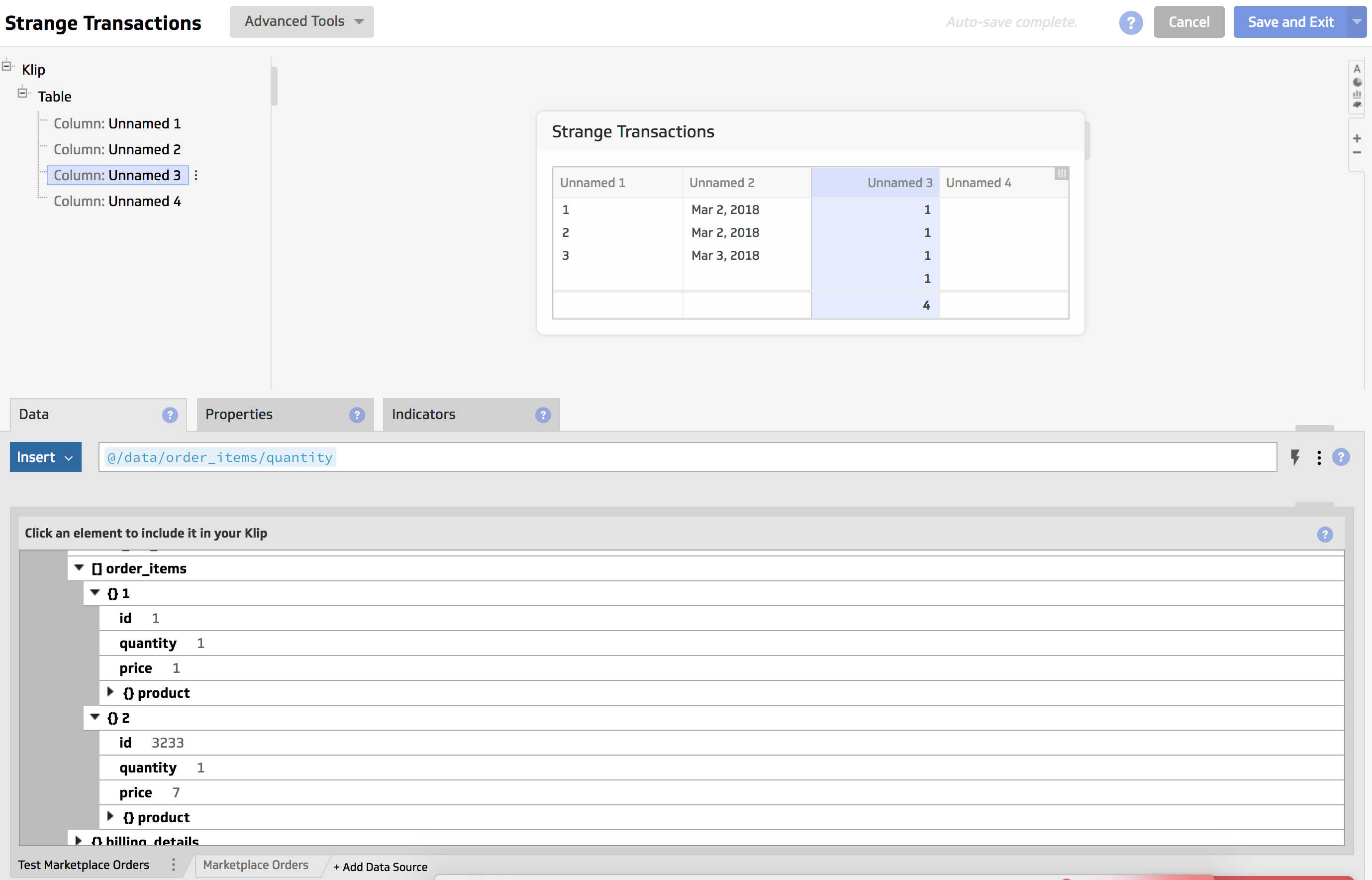Hi all,

I have a nested data source of orders in JSON format. Each object contains an array of order_items, and each order_item has a quantity. So, this is a nested JSON source.

See image:I am trying to sum the quantities within an order, but I can't seem to do this. As you can see from the image, if I use the selector "@/data/order_items/quantity;" it will create blank rows where there are multiple order_items in the object.

When I try to sum @/data/order_items/quantity, it returns the sum of all quantity and displays it only on the first row, when I want the sum of all quantities within a given row.

How do I get it to return the sum of the quantities for each row?

Thanks,

Leonard

•Kalyani Official comment

Hi -

You can actually repeat the data/id for each item under the order_items array. This would give you the same amount of records for data/id and order items so that you can group on the ids and sum the quantity under order_items.

The formula to repeat data ids would be -

column1 - /data/order_items/preceding-sibling::id

column 2- /data/order_items/quantity

Now, you can right-click and add a group action on column1 and use Sum aggregation on the second.

This would be a less complex and faster alternative solution to this situation.

Thanks,

Kalyani

•Leonard Teo

Just letting you all know the solution for future reference if people run into the same issue.

The trick is to use the MAP or MAPFLAT function and CONCAT to return aggregation results per object/row.

`MAPFLAT(  @/data/id,   "order_id",   SUM(    DATASOURCE(      "xx_insert_datasource_id_xx",       CONCAT("/data[id='",\$order_id,"']/order_items/quantity")    )  ))`

Let's walk through the above code:

1. MAPFLAT will iterate over each order ID, declare the variable "order_id"
2. For each order_id, we want the sum of the quantities at that order XPATH
3. Concat the order_id into the XPATH, refer to its datasource, and pass that into the SUM function.

•Josh Cohen-Collier

Hi Leonard!

Thanks for posting the solution here, and giving such a detailed explanation!

Best regards,
Joshua